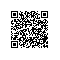# 06 回归算法 - 损失函数、过拟合欠拟合

### == 损失函数 ==

###### 0~1损失函数：

J(θ)=$begin{cases} 1,Y≠f(X)\ 0,Y=f(X)\ end{cases}$

###### 感知损失函数：

J(θ)=$begin{cases} 1,|Y-f(X)|>t\ 0,|Y-f(X)|≤t\ end{cases}$

###### 平方和损失函数：

J(θ)=$sum_{i=1}^m(h_θ(x^i) - y^i)^2$

###### 绝对值损失函数：

J(θ)=$sum_{i=1}^m|h_θ(x^i) - y^i|$

###### 绝对值损失函数：

J(θ)=$sum_{i=1}^m (y^ilogh_θ(x^i))$

### == 过拟合欠拟合 ==

###### 损失函数是评估模型好坏的一个指标，而一个模型坏的时候无非就是过拟合和欠拟合的问题。http://scott.fortmann-roe.com/docs/BiasVariance.html1、首先看最上方的曲线，表示总体的误差值。当模型欠拟合的时候总体误差值是最很大的，当模型的拟合度逐渐提高的时候，总体模型预测的误差值随之减小。但当拟合程度向过拟合发展的时候，虽然训练集上的拟合程度越来越高，但是在测试集上的误差会越来越大。
2、中间的曲线，表示偏差。随着拟合程度的增加，偏差会逐渐减小。
3、最下方的曲线，表示方差。随着拟合程度的增加，方差会逐渐增大。1、对于数据的预测该模型体现了一个明确的增长趋势。
2、虽然有误差但是误差始终保持在直线的上下两侧。

###### 误差 = 过拟合的方差+欠拟合的偏差2使用钉钉扫一扫加入圈子
+ 订阅